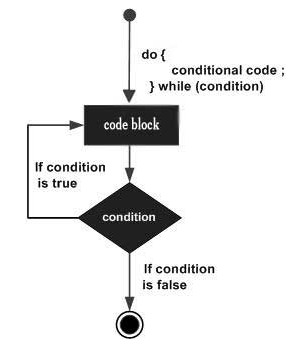×

Search anything:

# do while loop in Java

#### Java Software Engineering do while loopGet this book -> Problems on Array: For Interviews and Competitive Programming

Reading time: 15 minutes | Coding time: 2 minutes

A do-while loop is similar to a while loop, except the fact that it is guaranteed to execute at least one time. The do-while loop checks its condition at the bottom of the loop after one time execution of do and hence, is an exit control loop.

Syntax

``````do
{
statement(s);
}
while( condition );
``````

The condition appears at the end of the loop, so the statement(s) in the loop executes once before the condition is tested.

### Flow DiagramIf the condition is true, the flow of control jumps back up to do, and the statement(s) in the loop executes again. This process repeats until the given condition becomes false.

If the condition becomes false statement following the while statement will be executed.

Flow:

Step 1: Execute the code statements within while loop body
Step 2: Check condition
Step 3: If condition is True, go to Step 1. If condition is False, go to Step 4.
Step 4: Exit do while loop. Go to code statement immediately after do while loop

### Example

Program to print all natural number less than or equal to '6' and greater than 0 using do while loop.

``````class do_while_loop
{
public static void main (String[] args)
{
int i = 1;
do
{
System.out.println(i);
++i;
}while(i<6);
}
}
``````

Output:

``````1
2
3
4
5
``````

### Infinite loop using while loop

do While loop can run infinitely if the condition always evaluates to true. Setting the condition to true will result in infinite loop.

Keeping the condition empty will give compilation error.

``````class do_while_loop
{
public static void main (String[] args)
{
do{}while(true); // Infinite do while loop

do{}while(); // compilation error

do{}while(1==1); // Infinite do while loop
}
}
``````

### Benchmarking do while loop vs for and while loop

We will use the following code to perform this test:

``````class while_loop
{
public static void main (String[] args)
{
int count = 1;
long t1_1 = System.nanoTime();
while(count <= 1000000) count=count+1;
long t1_2 = System.nanoTime();

long t2_1 = System.nanoTime();
for(count=1; count <=1000000; count=count+1);
long t2_2 = System.nanoTime();

count = 1;
long t3_1 = System.nanoTime();
do{count=count+1;}while(count<=1000000);
long t3_2 = System.nanoTime();

System.out.println("Time taken by while loop: "+(t1_2-t1_1)+ " nanoseconds");
System.out.println("Time taken by for loop: "+(t2_2-t2_1)+ " nanoseconds");
System.out.println("Time taken by do while loop: "+(t3_2-t3_1)+ " nanoseconds");
}
}
``````

Output:

``````Time taken by while loop: 3602126 nanoseconds
Time taken by for loop: 1669825 nanoseconds
Time taken by do while loop: 7253640 nanoseconds
``````

Hence, we see, in terms of execution time for do while loop takes more time compared to while and for loop and hence, is the slowest looping statement. Hence, do while loop should be replaced by for loop or while loop whenever possible.

### do while > while > for

If execution time for for loop is taken as X, then:

for loop: X
while loop: 2X
do while loop: 4X#### OpenGenus Foundation

The official account of OpenGenus IQ backed by GitHub, DigitalOcean and Discourse

Improved & Reviewed by:

do while loop in Java